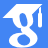• mira [at] ictp.acad.ro
••Orcid profile
•ResearcherID profile

Ph.D. in Mathematics (1997)

### Current position:

Former senior researcher (I) at “Tiberiu Popoviciu” Institute of Numerical Analysis

### Former research domains:

• Fixed point theory, linear and nonlinear operators
• The inverse and direct problem of Dynamics in Celestial Mechanics
• Combinatorics: complexity of finite and infinite words

## Applications of fibre contraction principle to some classes of functional integral equations

Abstract Let a<c<b real numbers, (B,|·|) a (real or complex) Banach space, H∈C([a,b]×[a,c]×B,B),K∈C([a,b]² ×B,B),g∈C([a,b],B),A:C([a,c],B)→C([a, c], B) and B:C([a,b],B)→C([a,b],B). In this paper we study the following functional integral equation, x(t)=∫_{a}^{c}H(t,s,A(x)(s))ds+∫_{a}^{t}K(t,s,B(x)(s))ds+g(t),t∈[a,b]. By…
Abstract We deal with the existence and localization of positive radial solutions for Dirichlet problems involving $$\phi$$-Laplacian operators in a ball. In particular, $$p$$-Laplacian and Minkowski-curvature equations are considered. Our…